In 2012, Chris Yakopcic co-authored a book chapter in the book titled Advances in Neuromorphic Memristor Science and Applications, featuring a chapter on memristor modeling in LTSpice. In this chapter, he does a wonderful review of the most common memristor models up to that point in time including LTSpice code and simulation results for simple sine wave driven hysteresis loops. Here, we repeat many of his simulations in order to gain a better understanding of the various models and to learn a few tricks about using LTSpice. Some of the basics for memristor modeling and setting up LTSpice was already published in a previous post. I would like to thank Chris Yakopcic for sending me all the LTSpice model files appearing in his book chapter and for sharing his techniques for running his simulations on LTSpice. For more of Chris’s work, check out his blog.

In this post I will run a few of Chris’s memristor model simulations in LTSpice followed our own implementation of our Knowm Mean Metastable switch model. And as usual, all model and simulation files appearing here can be found in our memristor-models-4-all project on github.

## LTSpice

In a previous post titled The Joglekar Resistance Switch Memristor Model in LTSpice, I already covered the basics of setting up LTSpice, memristor modeling in general and running a memristor model simulation in LTSpice. Here, I’ve adopted the methods of Yakopcic, so things are a tad bit different. For example, I added a third node to the memristor symbol, which is used to represent the internal state of the memristor, XSV. I didn’t connect it to anything and plotting the state is done by accessing the V(nc_01) variable. Another change from the previous post is that I link the memristor subcircuit not from the memristor symbol itself, but as a directive added to the simulation file (.asc). The Value attribute for the symbol needs to be updated however to match the subcircuit name. For each simulation there are two corresponding .plt files – one for I-V plots and one for time plots. LTSpice will look for a .plt file corresponding to the .asc file being run so what you need to do is remove the _IV and _T part of the complete .plt file name before running the simulation.

## Joglekar Window

Simulation Files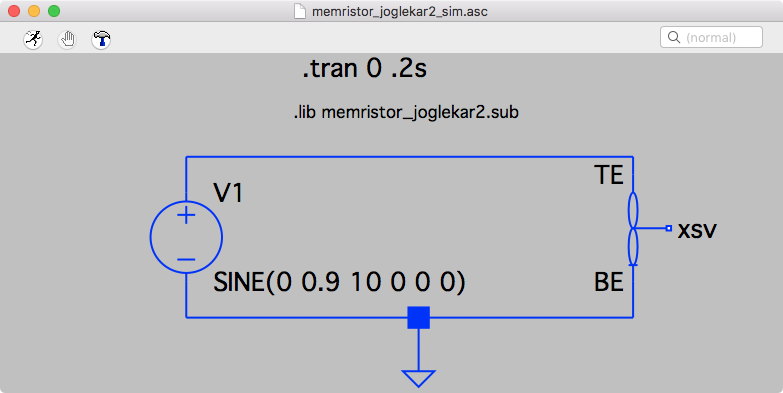LTSpice Circuit Joglekar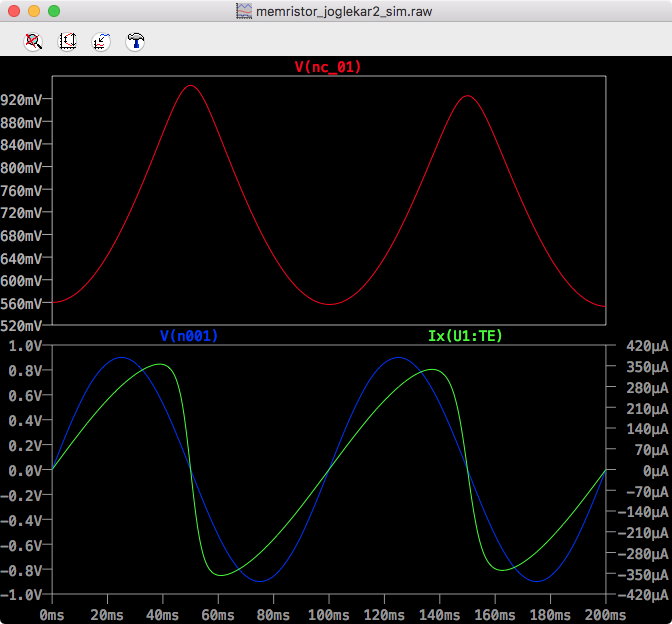LTSpice Joglekar Time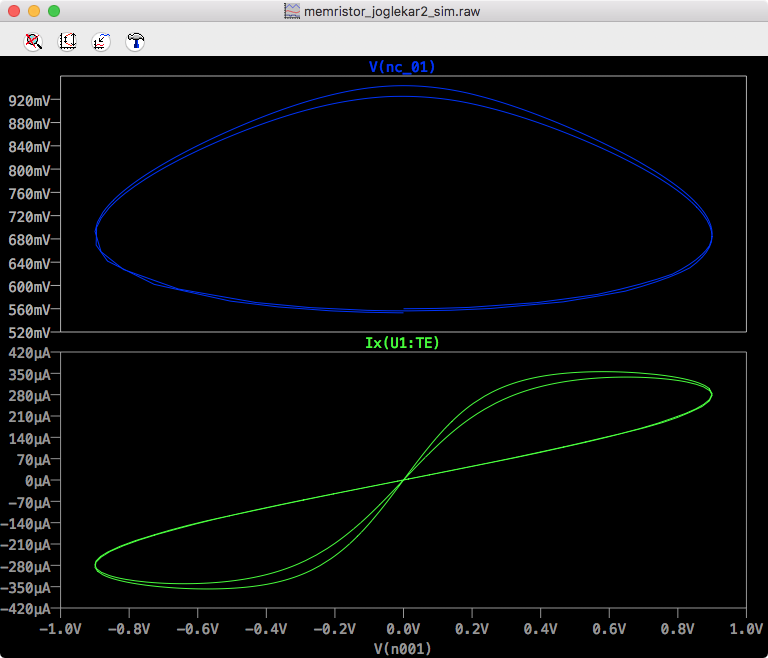LTSpice Joglekar IV

## Biolek Window

Simulation Files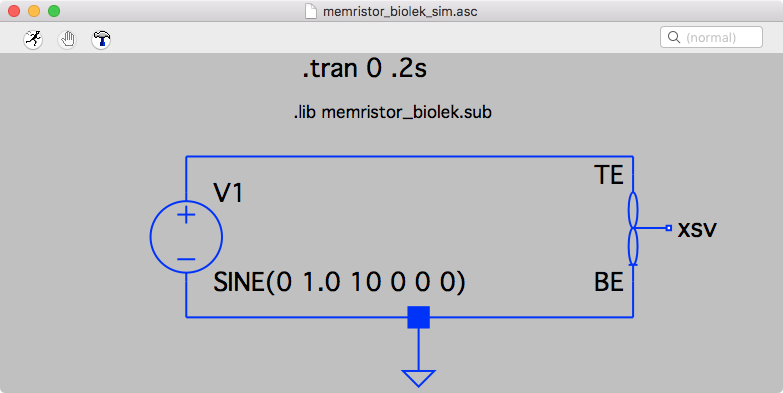LTSpice Circuit Biolek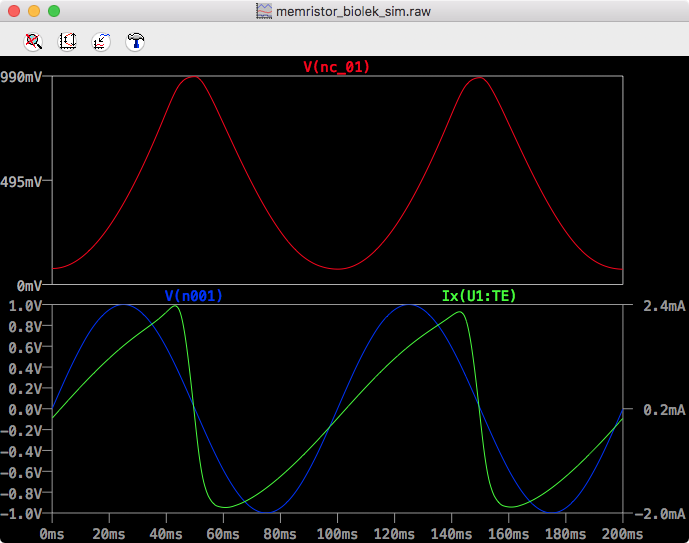LTSpice Biolek Time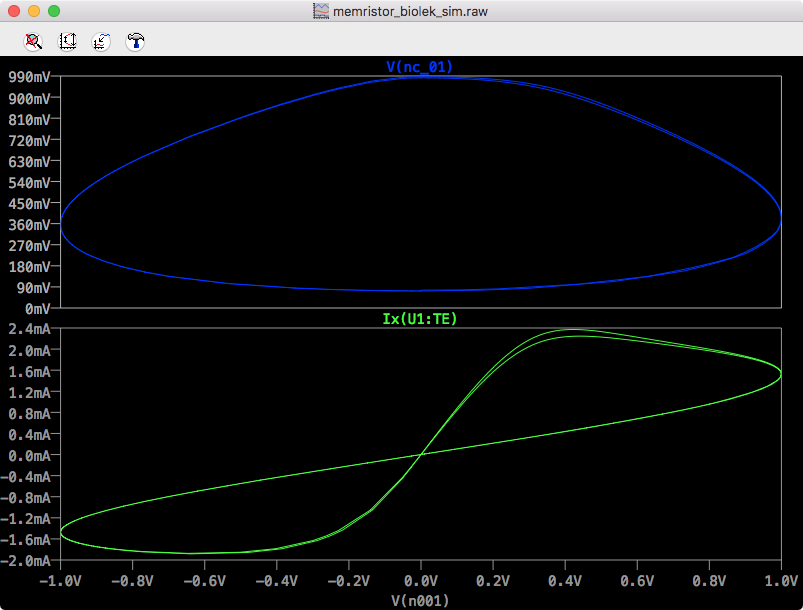LTSpice Biolek IV

## Yakopcic

Simulation Files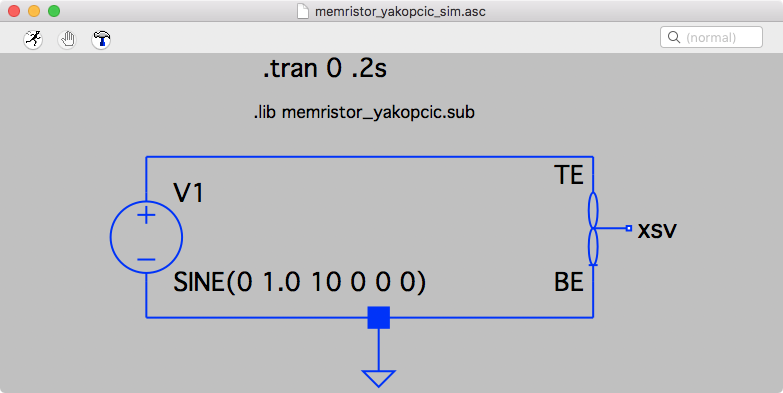LTSpice Circuit Yakopcic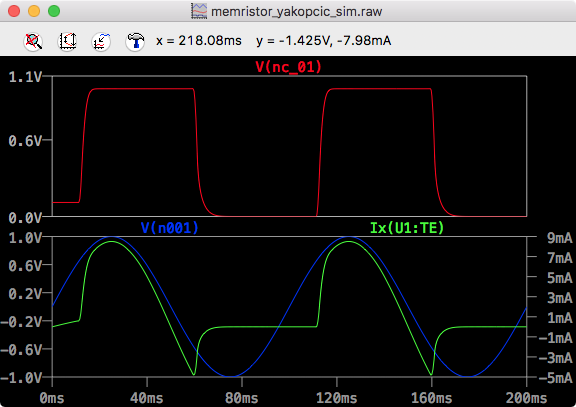LTSpice Yakopcic Time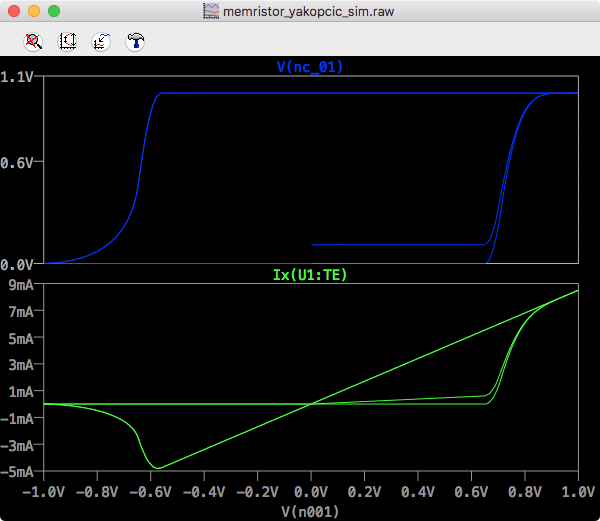LTSpice Yakopcic IV

## University of Michigan

Simulation Files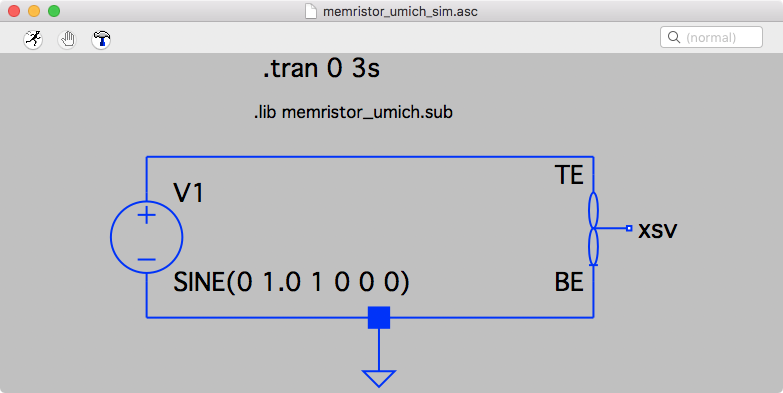LTSpice Circuit UMich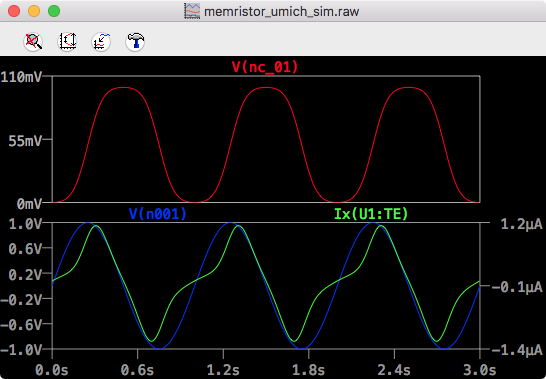LTSpice UMich Time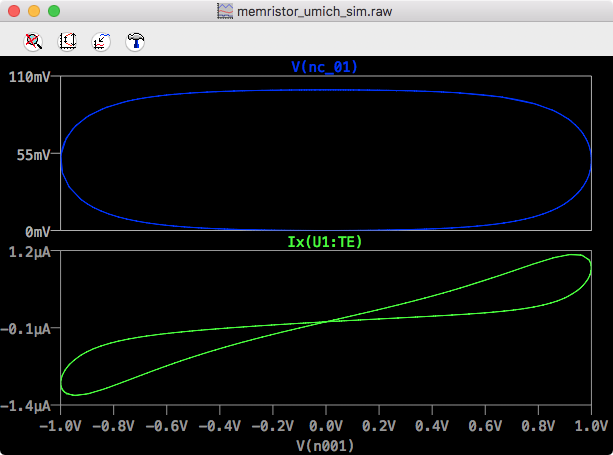LTSpice UMich IV

## Knowm

Simulation Files

Because this model does not appear in Yakopcic’s book chapter referenced above, I will include a quick review of the mode and the LTSpice subcircuit here.

## The Mean Metastable Switch Memristor Model

The mean metastable switch memristor model was previously described here, but I will summarize it again here for completeness. The model describes a memristor as a collection of metastable switches where each switch can be in either one or the other state. At any given time, the probability of switches switching state as a function of applied voltage is calculated.

The change in the number of switches, scaled between 0 and 1,$dX$ is:$dX = N_{OFF \rightarrow ON} - N_{ON \rightarrow OFF}$

If the probabilities$P_{OFF \rightarrow ON}$ and$P_{ON \rightarrow OFF}$ are defined as:$P_{OFF \rightarrow ON} = \alpha \frac{1}{{1 + {e^{ -\beta \left( {V - {V_{ON}}} \right)}}}}$$P_{ON \rightarrow OFF} = \alpha \left(1 - \left(\frac{1}{{1 + {e^{ -\beta \left( {V + {V_{OFF}}} \right)}}}}\right)\right)$

The number of switches switching state is thus:$N_{OFF \rightarrow ON} = P_{OFF \rightarrow ON} \cdot (1-X)$$N_{ON \rightarrow OFF} = P_{ON \rightarrow OFF} \cdot (X)$

. Therefore plugging everything in, also setting$\alpha = \frac{dT}{\tau}$:$dX = P_{OFF \rightarrow ON} \cdot (1-X) - P_{ON \rightarrow OFF} \cdot X$$dX = \frac{dt}{\tau} \frac{1}{{1 + {e^{ -\beta \left( {V - {V_{ON}}} \right)}}}} \cdot (1-X) - \frac{dt}{\tau} \left(1 - \left(\frac{1}{{1 + {e^{ -\beta \left( {V + {V_{OFF}}} \right)}}}}\right)\right) \cdot X$$dX/dt = 1/\tau \left[ \frac{1}{{1 + {e^{ -\beta \left( {V - {V_{ON}}} \right)}}}} \cdot (1-X) - \left(1 - \left(\frac{1}{{1 + {e^{ -\beta \left( {V + {V_{OFF}}} \right)}}}}\right)\right) \cdot X \right]$

The final step to complete the MMSS model description is to define the current as a function of X. We can break that definition down into two steps, first the conductance, followed by the current. The conductance as a function of X is$G = \frac{X}{ R_{ON} }+ \frac{1 - X}{R_{OFF}}$

Note that this describes a summation of conductances. Relating this to the real-world it tells us that our model is in fact 2 parallel resistors whose resistance values are coupled to each other via X.

For completeness, given a memristance value, for example$R_{init}$,$X = \frac{R_{ON} (R_{init} - R_{OFF} )}{ R_{init} (R_{ON} - R_{OFF})}$

Finally, by Ohm’s Law the current is$I = V \cdot G$

### Subcircuit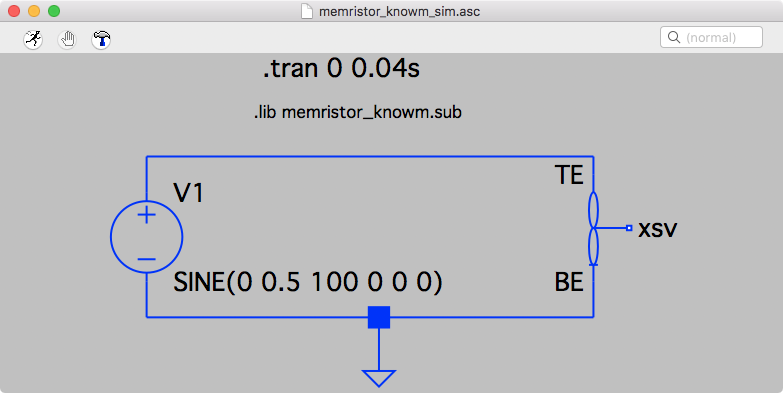LTSpice Circuit Knowm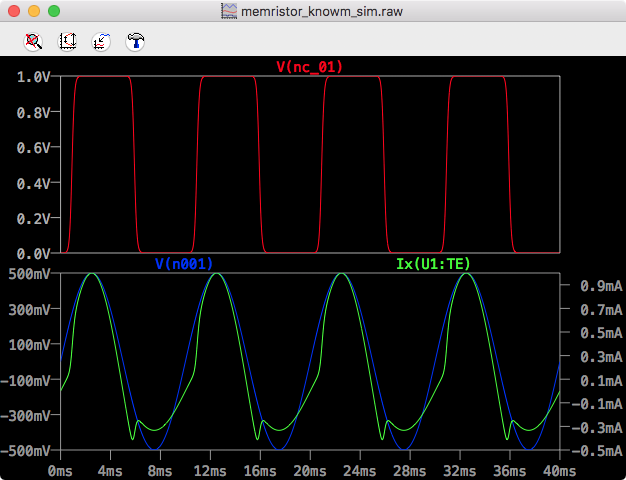LTSpice Knowm Time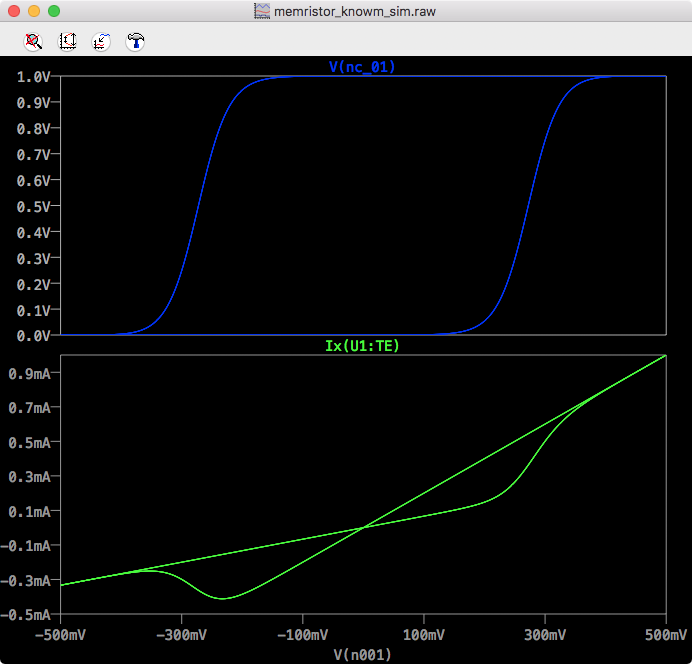LTSpice Knowm IV

## Conclusion

In this post I presented my simulation results taken more or less directly from Chris Yakopcic’s book chapter in the book titled Advances in Neuromorphic Memristor Science and Applications. I didn’t run all of the examples in the chapter, but doing so shouldn’t be hard at all. One of the motivations for running these simulations was to understand the basic mechanics of memristor modeling in LTSpice so that I could implement Knowm’s mean metastable switch memristor model, which I presented as the last simulation.

## Memristor Crossbars ...

Read part 1 of this series here Private Beta Ahoy! Well, I c...

## Memristor Crossbars ...

Time to Take the Next Step Memristor crossbars, and memristo...

## How to embed XChart ...

TL;DR [crayon-656e89b49b0b7080304747/] …and dont forge...